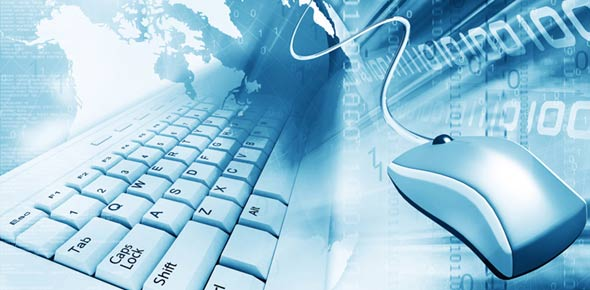# Final Examination In Computer Concepts And Fundamentals

8 Questions | Total Attempts: 764SettingsMultiple choice final examination for Computer Concepts and Fundamentals Course under Mr. Remacha.

• 1.
A criminal activity that is used by people to fraudulenty obtain personal information, such as usernames, passwords, cedit card details, and your social security information.
• A.

• B.

Hackers

• C.

Spyware

• D.

Phishing

• 2.
Most motherboards have how many IDE connectors?
• A.

One

• B.

Two

• C.

Three

• D.

One of the above

• 3.
Which part of the computer is rated by how many bits of information they can receive in one second?
• A.

Memory

• B.

Modem

• C.

Drive bay

• D.

Microprocessor

• 4.
Formulas are instructions that you enter to perform calculations.  For example, enter the number 350 in cell B1; enter the number 450 in cell B2; enter the formula =B1+B2 in cell B3.  The value 800 is displayed in cell B3.  If a value changes in cell B1 or B2, the formula will always add the two and show the correct sum in cell B3. If B1 is 25, B2 is 20, and B3 has the formula =B1-B2, what is the sum that will show in B3?
• A.

45

• B.

5

• C.

-5

• D.

None of the above

• 5.
Which of the following is not a hardware device?
• A.

Storage Devices

• B.

Output Devices

• C.

MSDOS

• D.

CPU

• 6.
Input refers to?
• A.

Programs

• B.

Commands

• C.

User responses

• D.

All of the Above

• 7.
When number signs (#####) are displayed in the cell, it means:
• A.

There are too many numbers in the cell.

• B.

The formula is wrong.

• C.

The decimal point is wrong.

• D.

All of the above

• 8.
The central processing unit is also called what?
• A.

Microprocessor

• B.

Motherboard

• C.

Power Supply

• D.

Control Unit

Related TopicsBack to top